Ch 6. Integrals Multimedia Engineering Math IndefiniteIntegral Area DefiniteIntegral FundamentalTheorem SubstitutionRule
 Chapter 1. Limits 2. Derivatives I 3. Derivatives II 4. Mean Value 5. Curve Sketching 6. Integrals 7. Inverse Functions 8. Integration Tech. 9. Integrate App. 10. Parametric Eqs. 11. Polar Coord. 12. Series Appendix Basic Math Units Search eBooks Dynamics Fluids Math Mechanics Statics Thermodynamics Author(s): Hengzhong Wen Chean Chin Ngo Meirong Huang Kurt Gramoll ©Kurt GramollMATHEMATICS - THEORY

This section introduces the Fundamental Theorem of Calculus.

Fundamental Theorem of Calculus

The concept of derivative and integral will be related by the Fundamental Theorem of Calculus. This theorem consists of two parts.

• Part one states:
If function f is continuous on interval between a and b, then the function g which is defined by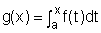a ≤ x ≤ b

is continuous on the [a,b], differentiable on (a, b), and g '(x) = f(x).
• Part two states:
If function f is continuous on the interval between a and b, then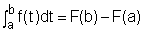(1)

where F is any antiderivative of f, that is F '(x) = f(x). The above equation can also be expressed as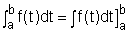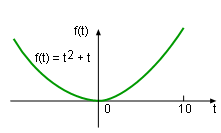Continuous Function f(t) = t2 + t

This theorem can be better understood by finding the result of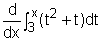.

The function t2 + t is a continuous function over the interval between 3 and x. According to the first part of Fundamental Theorem of Calculus, the derivative of this function is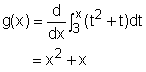In order to double check the answer,can be determined by an alternative method. First, use the second part of Fundamental Theorem of Calculus to find the antiderivative of on the interval [3, x].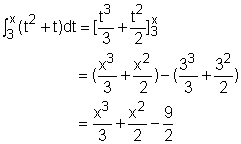Function Antiderivative ∫cf(x)dx cF(x) + c ∫xndx   (n ≠ -1) xn+1/(n + 1) + c ∫(f(x) + g(x))dx F(x) + G(x) + c ∫cosxdx sinx + c ∫sinxdx -cosx + c ∫sec2xdx tanx + c ∫secxtanxdx secx + c ∫cscxcotxdx -cscx + c ∫csc2xdx -cotx + c

Next, take the derivative of the result gives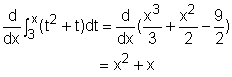It is clear that both methods give the same results. In order to use the Fundamental Theorem of Calculus effectively, important formulas of antiderivative are given in the table on the left.